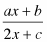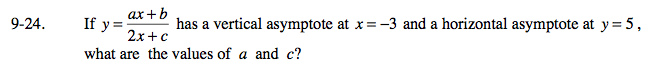### Home > AC > Chapter 16 > Lesson 16.9.1.2 > Problem9-24

9-24.
1. If y =has a vertical asymptote at x = −3 and a horizontal asymptote at y = 5,

2. what are the values of a and c? Homework Help ✎A vertical asymptote occurs due to division by zero.

$2(-3) + c = 0$

A horizontal asymptote of 5 means that the dominant term is in the numerator and the denominator. Therefore:

$\frac{a}{2} = 5$